# Looking at Fertility in R

Fertility is something people don’t typically discuss openly in the US, which isn’t a surprise because it is an incredibly personal topic. In fact, it’s really difficult to even write a blog post about, I wrote this over a year ago and I’m only getting around to posting it now. It took us roughly 7 months to conceive a baby, and I’m proud to say we now have a happy baby boy!However, every negative pregnancy test you see takes an emotional toll on you (and can even put strain on some marriages). During that time, I found that research online wasn’t extremely helpful. My wife and I found it relatively difficult to find answers to two very important questions:

• What are the odds of a couple conceiving each month?
• How much of a factor does age play?

I need to start this off by saying, I am not a doctor (nor do I play one on TV). In fact, I’m just going to start my exploration of this topic by first reading some blogs on the topic. This isn’t typically a great option, but then again, I’m writing a blog as well… What could go wrong, a blog based off of other blogs which might be discussed in another blog? I digress.

#### What difference does a woman’s age make?

According to a post on Today’s Parent by Alex Mlynek, an OB-GYN doctor stated that your chances of conceiving each cycle are:

• 25% to 30% if you’re under 35 years old
• 15% if you’re 35 to 39
• 5% if you’re 40 to 42
• 2% at 43
``````single_cycle = tibble(
age = factor(c("< 35", "< 35", "35 - 39", "40 - 42", "43"), levels = c("< 35", "35 - 39", "40 - 42", "43")),
average = c(0.25, 0.30, 0.15, 0.05, 0.02))

p = ggplot(single_cycle, aes(x = age, y = average))
p + geom_point(size = 3) +
geom_text(aes(label = scales::percent(average)), nudge_x = 0.15) +
geom_line() +
ggtitle('Single-Cycle Chance of Conception by Age') +
xlab('Age') +
ylab('') +
scale_y_continuous(labels = scales::percent, limits = c(0, 0.4)) +
ggthemes::theme_economist()``````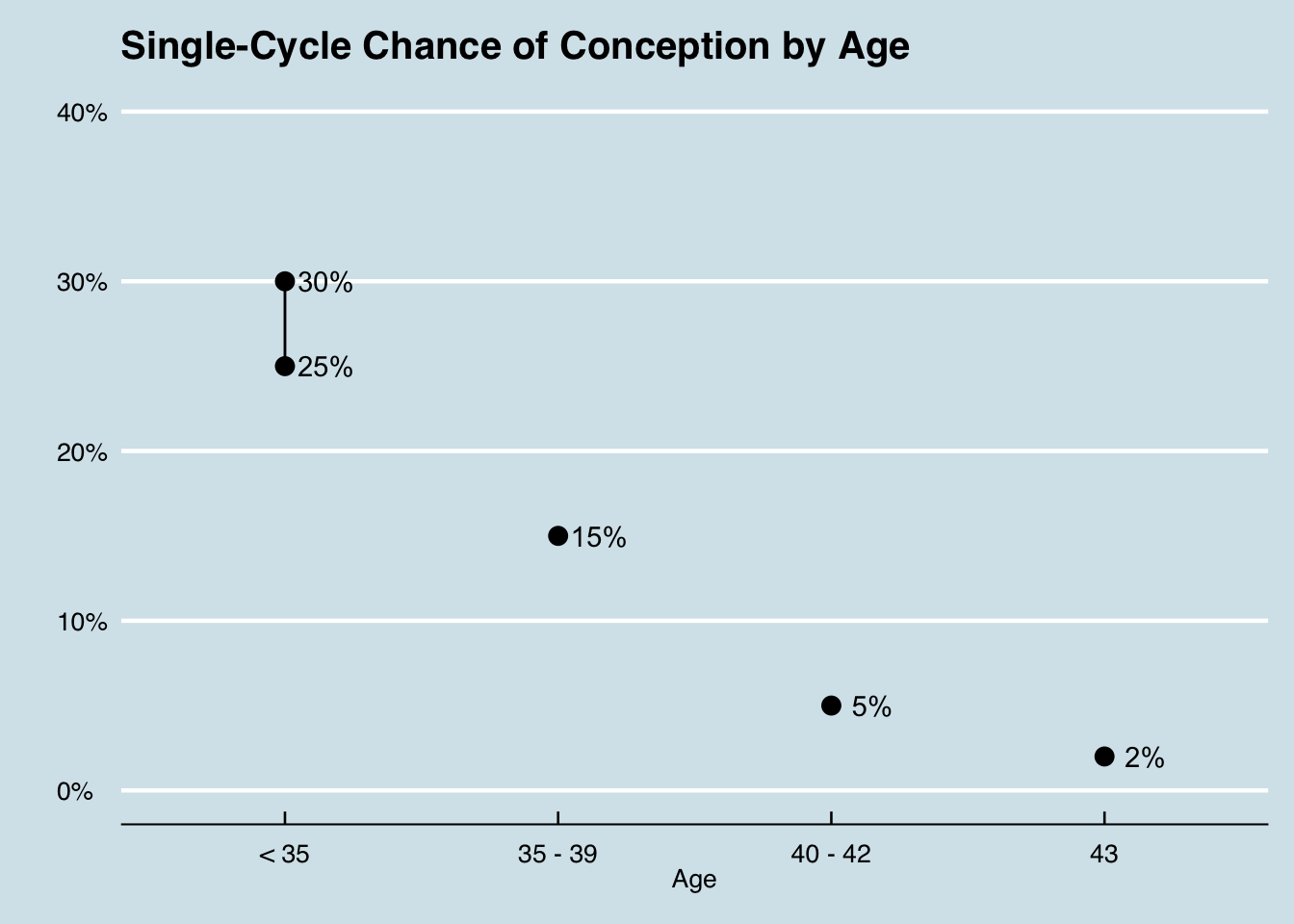#### What are the odds over the course of many months?

The article cites a 2003 study in the journal Human Reproduction of 346 women ages 20 to 44 who tracked ovulation cycles and timed intercourse that improved chances of conception:

• It led to a 38% chance of conception within one cycle
• 68% within three cycles
• 81% within six cycles
• 92% chance within twelve cycles
``````cycles = tibble(
cycle = factor(c('One', 'Three', 'Six', 'Twelve'), levels = c('One', 'Three', 'Six', 'Twelve')),
average = c(0.38, 0.68, 0.81, 0.92))
p = ggplot(cycles, aes(x = cycle, y = average))
p + geom_point(size = 3) +
geom_text(aes(label = scales::percent(average)), nudge_x = 0.15) +
ggtitle('Chance of Conception by Cycle (while Tracking Ovulation)') +
xlab('Cycle') +
ylab('') +
scale_y_continuous(labels = scales::percent, limits = c(0, 1)) +
ggthemes::theme_economist()``````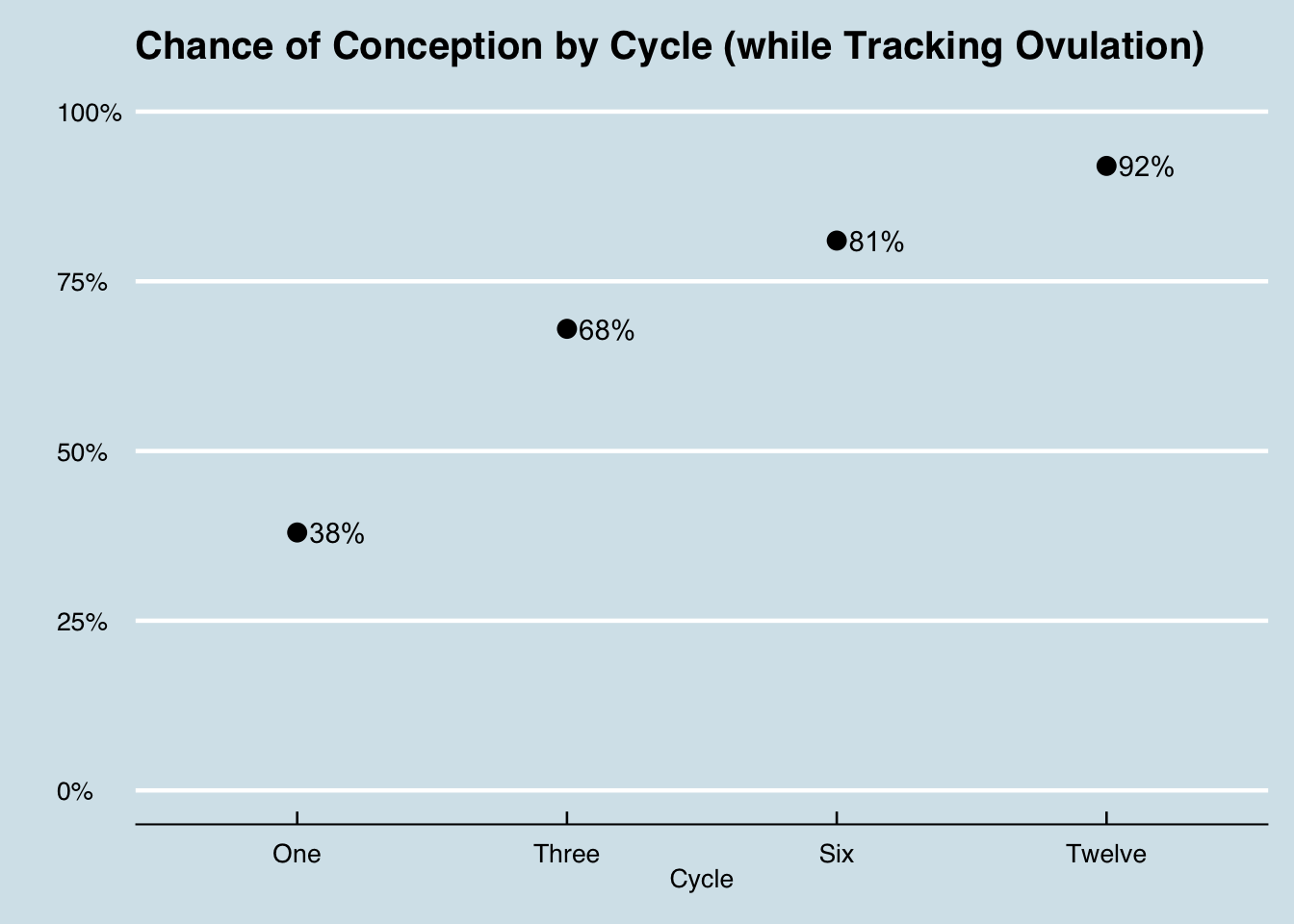Multiple articles (including the one above) indicate 85% of women will get pregnant within one year of trying and “if you have been trying for a year without conceiving and are under 35, she suggests that you seek a fertility consultation. If you’re 35 or older, you should seek help after just six months of trying because egg quality declines and medical conditions become more prevalent as we age, so the likelihood that you may need some fertility help is higher.”

As with almost everything in life, these numbers aren’t perfect and many factors affect the chances of getting pregnant. However, there seems to be a consensus that age is a major factor in determining the chances of conception.

### Now it’s time for some interpolation based off of absolutely no knowledge of the subject matter or understanding of the data itself!

We live in a world in which finding data is easier than ever before. It is absolutely awesome for a lot of reasons but can really lead to strong issues. Can you spot the problem with what I’m doing in the following examples?

``````cycles = tibble(
cycle = 1:12,
average = c(0.38, NA, 0.68, NA, NA, 0.81, NA, NA, NA, NA, NA, 0.92))

p = ggplot(cycles, aes(x = cycle, y = average))
p + geom_point(size = 3) +
ggtitle('Chance of Conception by Cycle') +
xlab('Cycle') +
ylab('') +
scale_x_continuous(limits = c(0,12), breaks = 1:12) +
scale_y_continuous(labels = scales::percent, limits = c(0, 1)) +
geom_smooth(method='loess') +
ggthemes::theme_economist()``````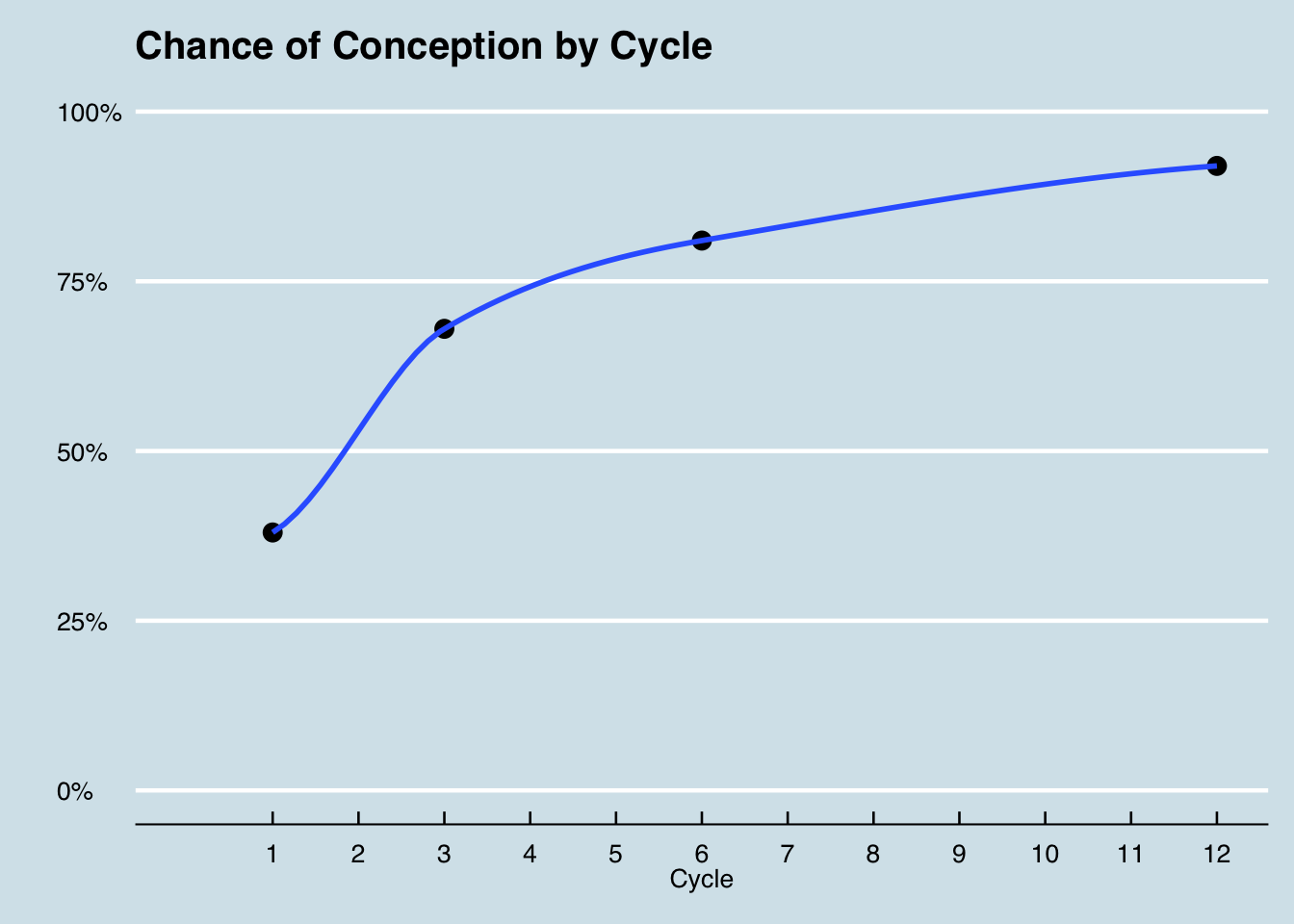Here’s the graph above represented as a table:

``````cycle = c(1, 3, 6, 12)
avg = c(0.38, 0.68, 0.81, 0.92)
lo = loess(avg ~ cycle)
lo12 = predict(lo, 1:12)
cycles_predicted = tibble(
cycle = 1:12,
predicted_average = round(lo12, 2)
)
ggtexttable(cycles_predicted, rows = NULL)``````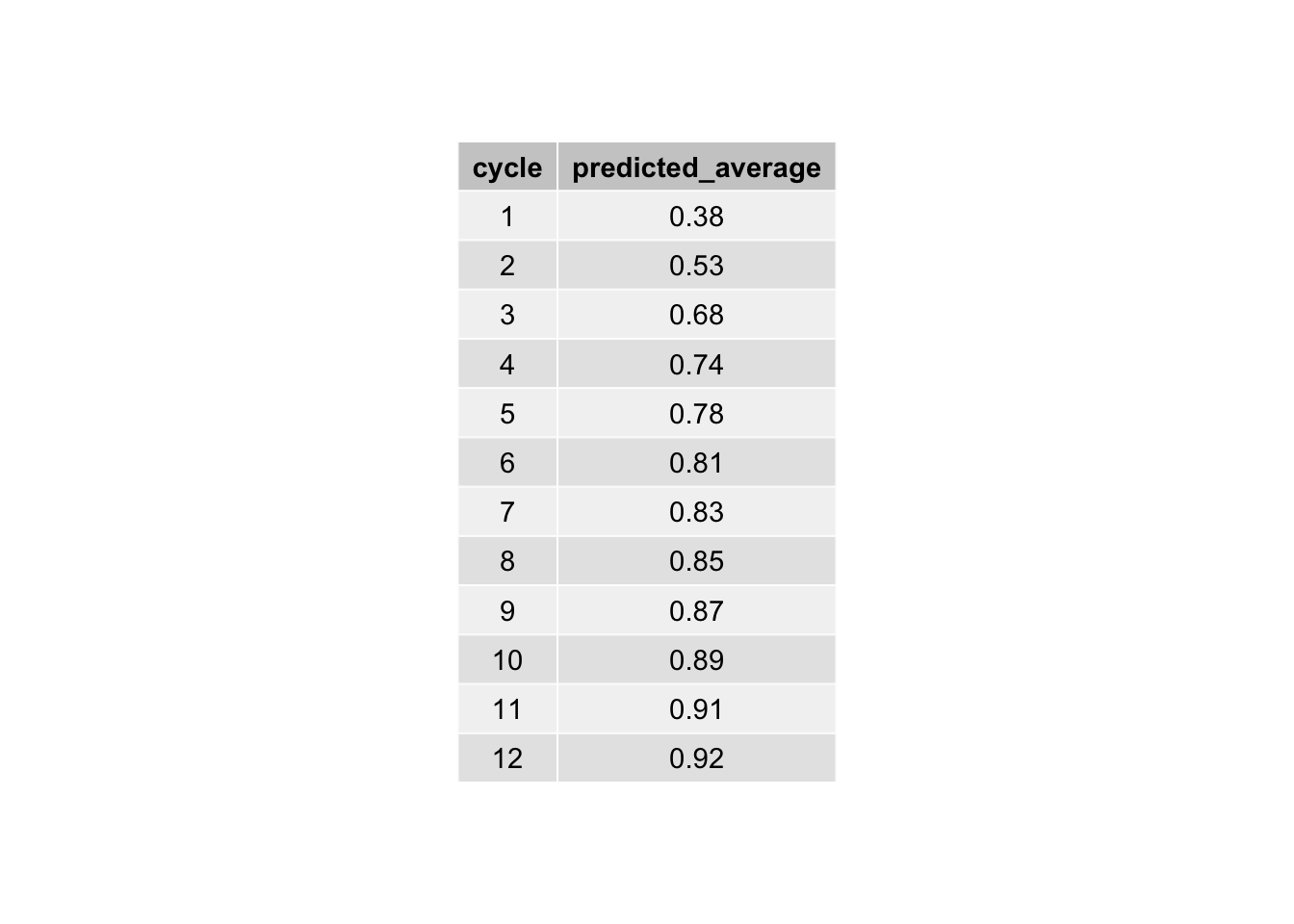Here’s the percent difference compared to the “baseline” monthly conception rate of 27.5% for women under 35:

``````#using .275 for simplicity
age = factor(c("< 35", "35 - 39", "40 - 42", "43"), levels = c("< 35", "35 - 39", "40 - 42", "43"))
benchmark = 0.275
avg_by_age = c(0.275, 0.15, 0.05, 0.02)

single_cycle = tibble(
age = age,
average_by_age = avg_by_age) %>%
mutate(age_effect = round((average_by_age - benchmark) / benchmark, 2))

ggtexttable(single_cycle, rows = NULL)``````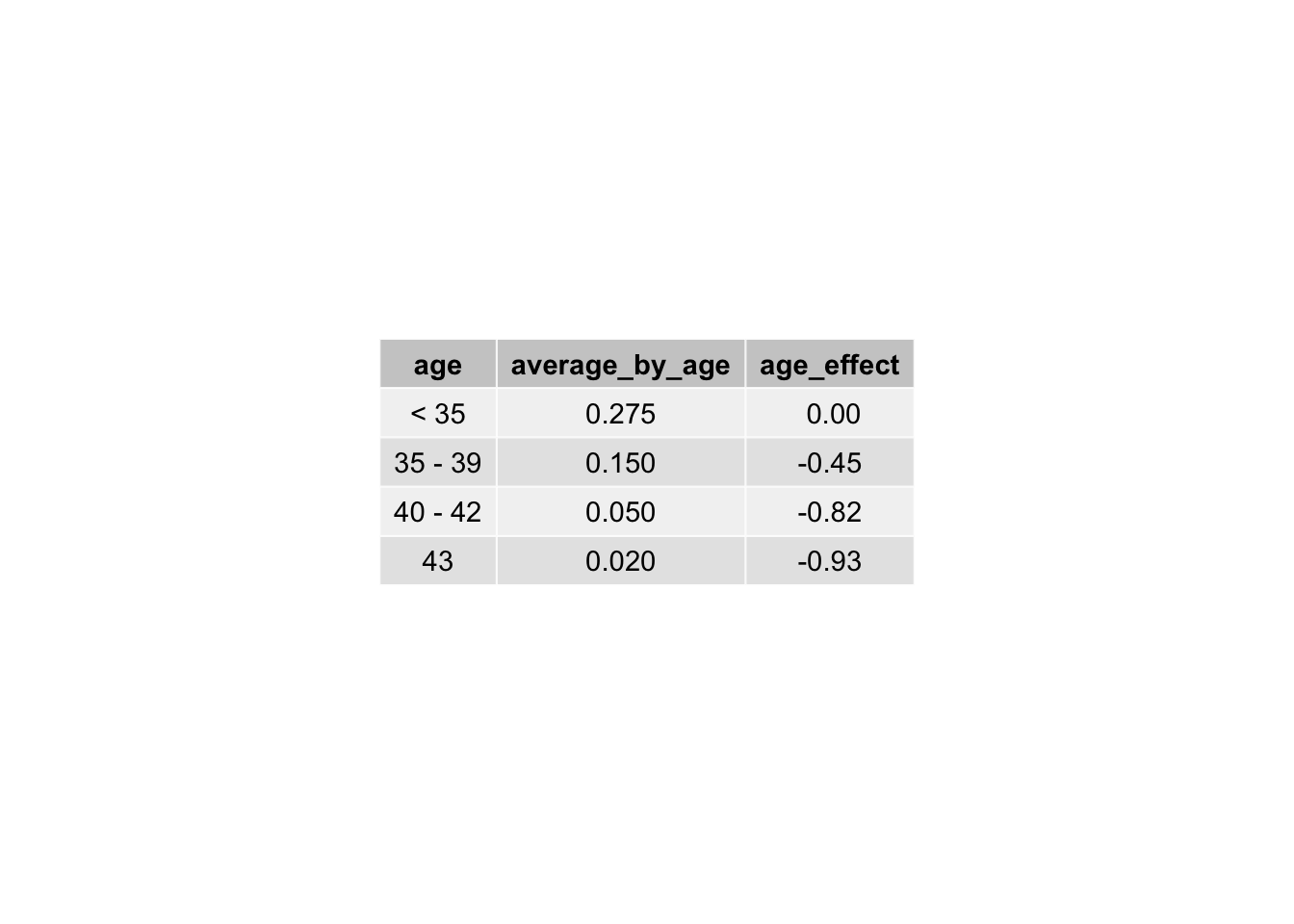Here is the same curve being fit to each age range:

``````age_prediction_plot = age_predictions %>%
select(-predicted_average) %>%
gather(age, predicted_average, -cycle)
p = ggplot(age_prediction_plot, aes(x = cycle, y = predicted_average, col = age))
p + geom_line(size = 1.5) +
ggtitle('Multiple-Cycle Chance of Conception by Age') +
xlab('Cycle') +
ylab('') +
scale_x_continuous(limits = c(0,12), breaks = 1:12) +
scale_y_continuous(labels = scales::percent, limits = c(0, 1)) +
ggthemes::theme_economist()``````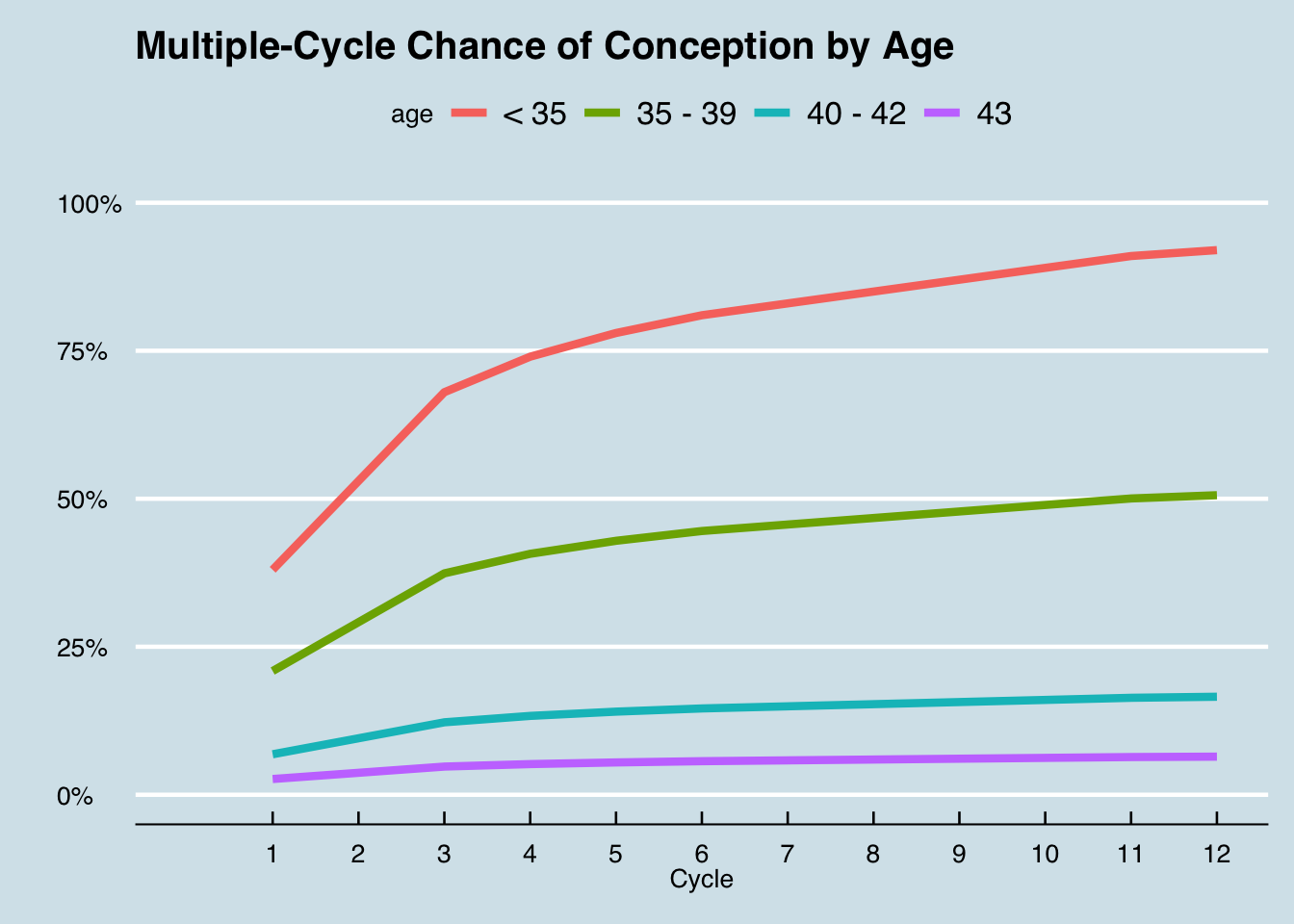Here is the chart above depicted as a table:

``````age_predictions = cycles_predicted
for(i in age){
age_predictions = age_predictions %>%
mutate(!!i := predicted_average * (1 + single_cycle\$age_effect[single_cycle\$age == i]))
}

ggtexttable(age_predictions %>% select(-predicted_average), rows = NULL)``````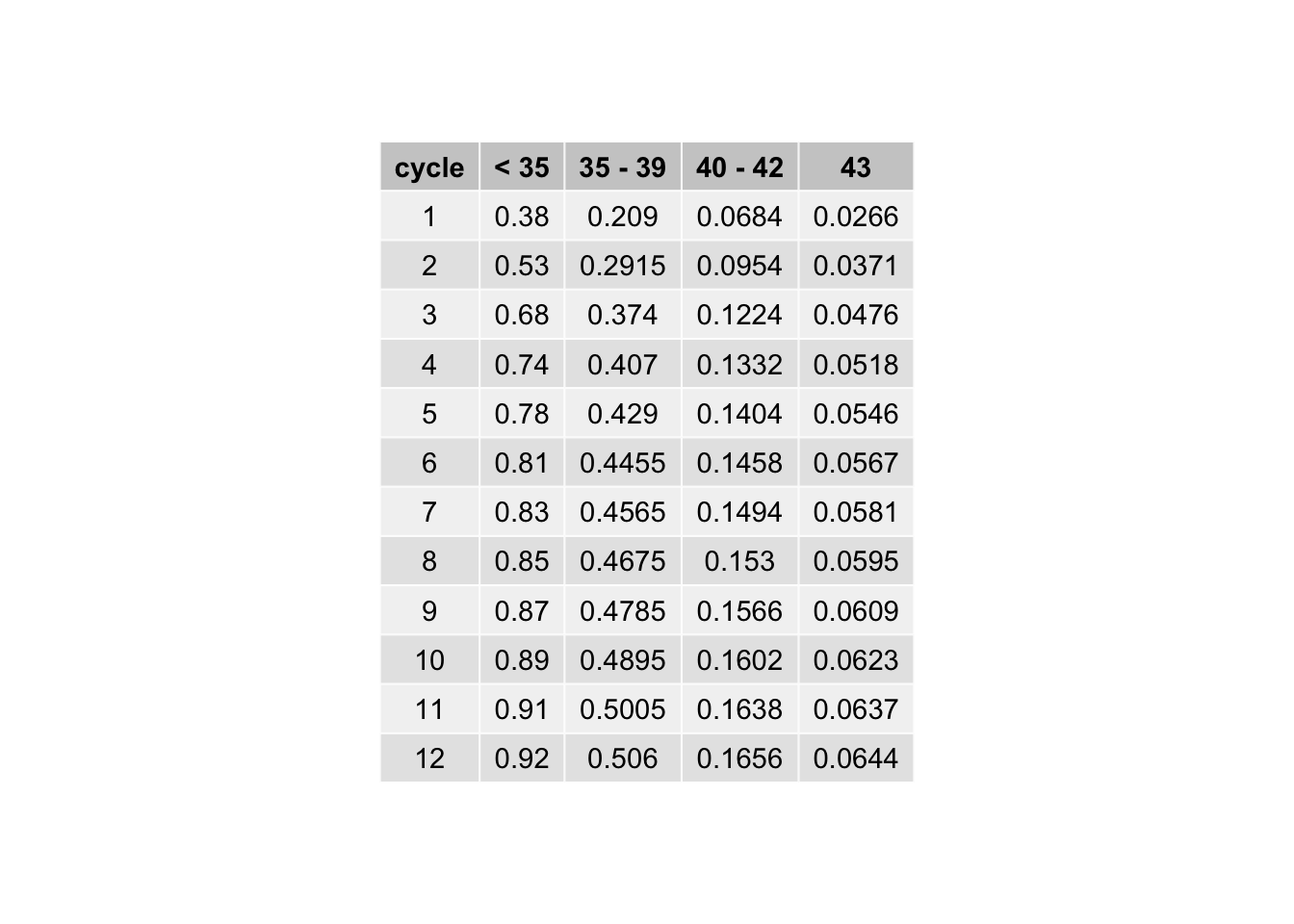I hope you were able to spot the problems, if not, I’ll be back to give you a hint in a little while.

Thank you so much for reading, I’m sorry it’s been a while since I’ve written anything. I’m making a conscious effort to write more in the upcoming months. As always, my code is available on GitHub